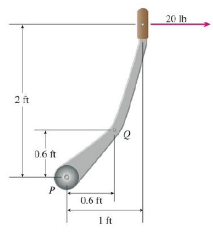Chapter 12.4, Problem 40E

Chapter
Section
Textbook Problem

# (a) A horizontal force of 20 lb is applied to the handle of a gearshift lever as shown. Find the magnitude of the torque about the pivot point P.(b) Find the magnitude of the torque about P if the same force is applied at the elbow Q of the lever(a)

To determine

To find: The magnitude of the torque about pivot point P.

Explanation

Given:

|F|=20lb .

Formula:

Write the expression to find magnitude of the torque.

|τ|=|r||F|sinθ (1)

Here,

r is the vector position,

F is the force, and

θ is the total angle between r and F .

From figure, the position vector (r) at point P to the handle is 1,2 .

The magnitude of the position vector (|r|) at vector 1,2

|r|=(1ft)2+(2ft)2=1+4ft=5ft

The total angle between force (F) and position vector (r) is,

θ=tan1(21)=tan1(2)=63

(b)

To determine

To find: The magnitude of the torque about point P if the same force is applied at the elbow Q of the lever.

### Still sussing out bartleby?

Check out a sample textbook solution.

See a sample solution

#### The Solution to Your Study Problems

Bartleby provides explanations to thousands of textbook problems written by our experts, many with advanced degrees!

Get Started

#### Evaluate the integral, if it exists. 01y(y2+1)5dy

Single Variable Calculus: Early Transcendentals, Volume I

#### Write each of the following in radical form. (a) x3/7 (b) x1/2 (c) x3/2

Mathematical Applications for the Management, Life, and Social Sciences

#### Show that ddx1+tanhx1tanhx4=12ex/2.

Single Variable Calculus: Early Transcendentals

#### The third partial sum of is:

Study Guide for Stewart's Multivariable Calculus, 8th